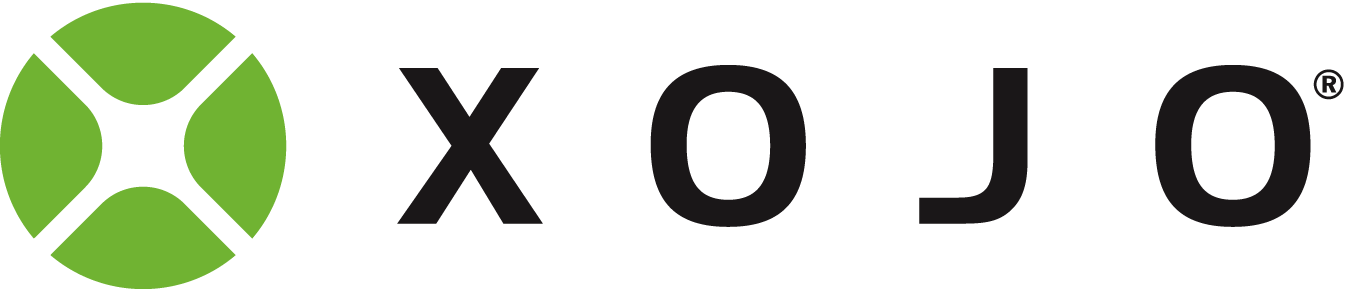Canvas MouseMOve

H there,

I fill a Canvas with squares (it’s a map with primes):

Var BlockWidth As Integer = 2
Var BlockHeight As Integer = 2
Var Space As Integer = 1

NumberTeller = 1
PrimeCounter = 0
PrimeMap = New Picture(CanvasWidth,CanvasHeight)

For X As Integer = 0 To CanvasHeight Step (BlockWidth + Space)
For Y As Integer = 0 To CanvasWidth Step (BlockWidth + Space)
If IsPrime(NumberTeller) Then
PrimeMap.Graphics.DrawingColor = PrimeColor
PrimeCounter = PrimeCounter + 1
Else
PrimeMap.Graphics.DrawingColor = NonPrimeColor
End If

PrimeMap.Graphics.FillRectangle(Y,X, BlockWidth,BlockHeight)
NumberTeller = NumberTeller + 1
Next
Next

In the Canvas.MouseOver event, how can I determine on which square the mouse pointer actually is?

do the maths in reverse in the mouse move event

something like this (untested)

leftpos =  x\(blockwidth + space)
toppos = y\(blockwidth + space)

rows = canvasheight\(blockwidth + space)
number  = leftpos * rows + toppos

note your code loops have x for vertical , and y for horizontal - don’t confuse these with x and y parameters

Hi,

thanks. Yes I reversed X and Y. I will try your suggestion.

Alex

Works!, I changed X and Y:

Var LeftPos As Integer = y\(Blockwidth + Space)

Var TopPos As Integer = x\(Blockwidth + Space)

Var Rows As Integer = Me.height\(Blockwidth + Space)
Var Number As Integer  = (Leftpos * Rows) + Toppos
PlayCanvas.Tooltip = Number.ToString
Forum for Xojo Programming Language and IDE. Copyright © 2021 Xojo, Inc.